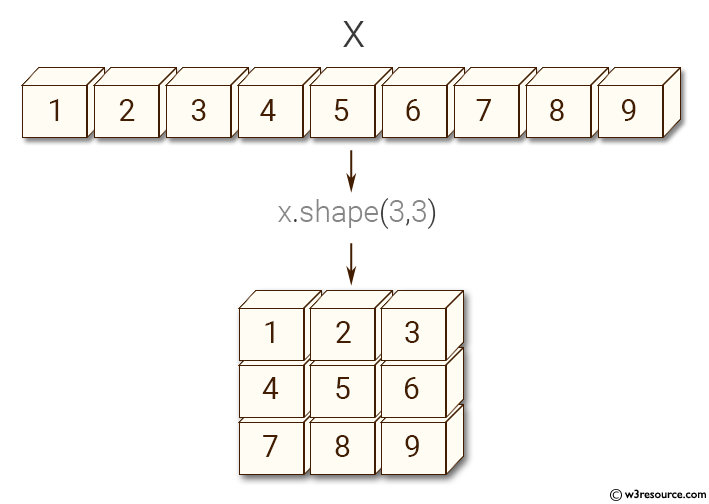﻿ NumPy: Change the dimension of an array - w3resource# NumPy: Change the dimension of an array

## NumPy: Array Object Exercise-35 with Solution

Write a NumPy program to change the dimension of an array.

Pictorial Presentation:Sample Solution:-

Python Code:

``````import numpy as np
x = np.array([1, 2, 3, 4, 5, 6])
print("6 rows and 0 columns")
print(x.shape)

y = np.array([[1, 2, 3],[4, 5, 6],[7,8,9]])
print("(3, 3) -> 3 rows and 3 columns ")
print(y)

x = np.array([1,2,3,4,5,6,7,8,9])
print("Change array shape to (3, 3) -> 3 rows and 3 columns ")
x.shape = (3, 3)
print(x)
```
```

Sample Output:

```6 rows and 0 columns
(6,)
(3, 3) -> 3 rows and 3 columns
[[1 2 3]
[4 5 6]
[7 8 9]]
Change array shape to (3, 3) -> 3 rows and 3 columns
[[1 2 3]
[4 5 6]
[7 8 9]]
```

Python Code Editor:

Have another way to solve this solution? Contribute your code (and comments) through Disqus.

What is the difficulty level of this exercise?

Test your Python skills with w3resource's quiz

﻿

## Python: Tips of the Day

Set comprehension:

```>>> m = {x ** 2 for x in range(5)}
>>> m
{0, 1, 4, 9, 16}
```